Share

# The Evolution of Maths From Antiquity to Modernity

By Jon, published on 31/03/2018 > > > The History of Maths

Mathematics is the science of description, demonstration and calculation, according to mathematician Ronald Brown.

Its many branches include disciplines like geometry, which describes lengths, areas and angles; arithmetic, or number theory; mechanics, describing the motion of bodies under the influence of a system of forces and stochasticity, describing random phenomena.

Here, we’re going to look at an overview of the major advances in maths over time.

Do you know where and when mathematics originated? Read on as we find out.

## The Birth of Maths in Antiquity

The ancient Egyptians were among the first to use mathematics (so yes, the first maths tutors were Egyptian, too). Excavations in Mesopotamia in the 19th Century unearthed Sumerian clay tablets written in cuneiform, dating either from the Babylonian dynasty (1800-1500 BC) or the ancient Greek period (600-300 BC).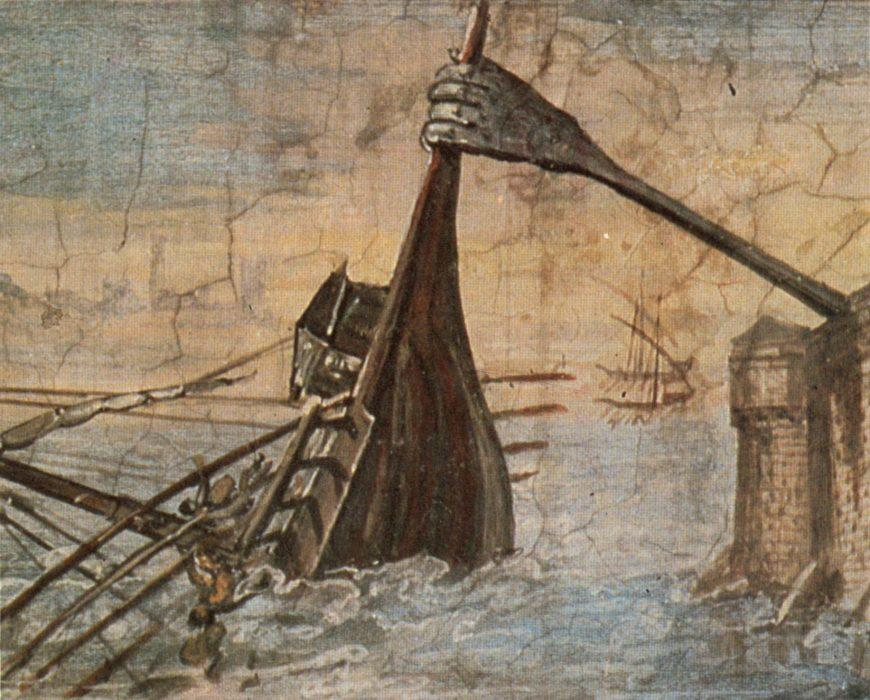The Claw of Archimedes (Source: wikipedia.org – Giulio Parigi)

These unique objects attest to the writers’ ability to solve quadratic equations. Their contents deal with commerce, specifically the buying and selling of bags of grain and slaves.

Well-known Greek philosophers, among whom Pythagoras, Thales and Plato, first developed and applied arithmetic, now also called number theory.

At that time, mathematics would travel from all over the empire in order to reach Alexandria and its famous school.

In the 4th century BC, Diophantus of Alexandria pioneered the discipline of algebra.

Later, elementary mathematics was thus born with Euclid, Archimedes of Syracuse and Apollonius of Perge. Euclid is the author of the Elements, the second best-selling book worldwide, after the bible, as well as volumes devoted to Euclidian geometry, with its five postulates, including the famous “any straight line segment can be extended indefinitely in a straight line”, which was later to serve as a reference point in geometry when that discipline appeared centuries later.

Archimedes, a great scientist from Sicily, also greatly contributed to geometry, including the approximation of pi, the Quadrature of the Parabola and the Archimedean spiral. In terms of statics, he was most interested in the principle of lift, in the form of pulleys, and in the creation of war machines, like the catapult, in order to study the action of forces.

He is mainly known for his work on buoyancy.

Find a maths tutor in no time on Superprof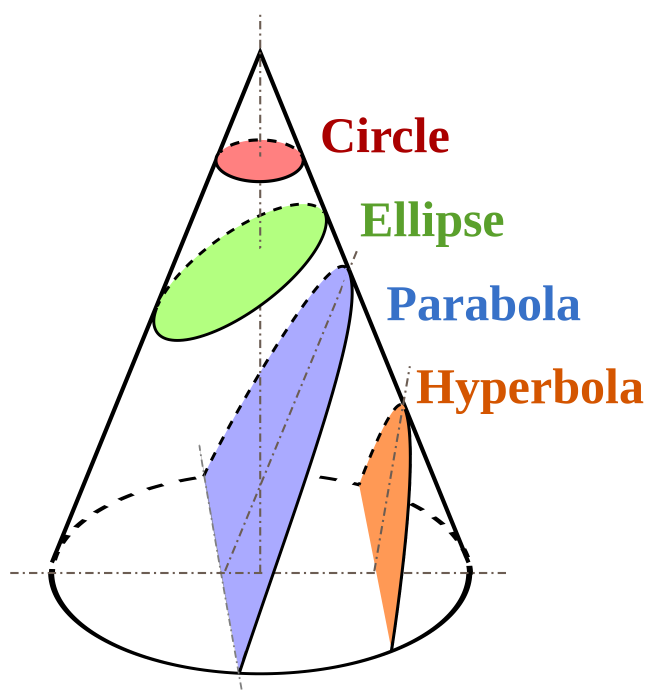Conic sections, pioneered by Apollonius of Perga (Source: commons.wikimedia.org)

Did you know that he also designed the biggest ship of the age, the Syracusia, and that it is to him that we owe the expression “Eureka”, which means “I’ve fount it!”?

Apollonius of Perga, on the other hand, is known for his work that led to our understanding of conic sections. It to him that we owe the terms ellipse, parabola and hyperbola. His work became important for astronomy, in the calculation of eccentric orbits and in understanding the motion of the planets.

Later, Ptolemy, Pappus and Hipparchus laid down the foundations of what was to become trigonometry: the branch of maths dealing with the relationships between angles and distances in triangles.

The concept of zero was independently invented by a number of civilisations, including India, from where it made its way into Western and Arabic mathematics.

Discover seven surprising connections between art and maths!

## Mathematics from the Middle Ages to 1900

In the 9th century, Arab mathematicians like Al Khwarizmi compiled Greek and Indian knowledge on mathematics. The introduction of Arab numerals in the 11th century marks the time at which mathematics began to emerge from obscurity, and the great thinkers began to become more recognised.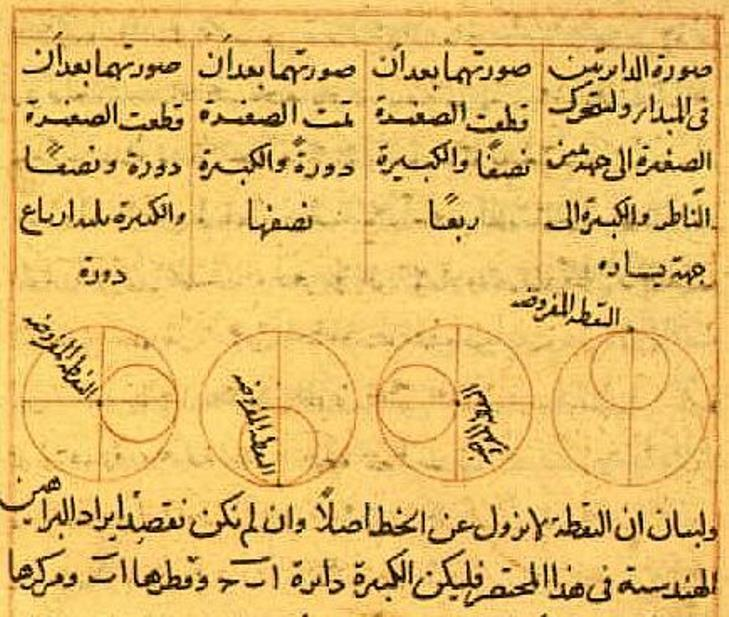Nasir al-Din Tusi’s Tusi couple, designed to model the motion of planets (Source: .wikipedia.org)

From the 12th century, the mathematical sciences rose to prominence, even in favour of rhetoric, grammar and logic. In Spain where Arabic sciences were taught, great scholars like Averroes and Avenzoar rose to fame.

In the fifteenth century, the system of addition and subtraction, and the symbols + and -, were first proposed by Johannes Widmann, born in Eger in what was then part of the Holy Roman Empire. The work of French mathematician François Viète made great strides in transforming algebra into its modern form, through the introduction of letters, to represent known or unknown quantities and to simplify equations.

In one colourful anecdote, Viète managed to break a 500 character cypher used by the Spanish, which led to the settlement of the French Wars of Religion – and the accusation by the Spanish crown of having used magical powers!

The 17th century is the golden age of mathematics. One of the most enduring tales of this period is that of Newton and the apple, which led to the theory of gravity. Here are some other important advances:

• Neper’s logarithms (1614) Also known as hyperbolic logarithms because they represent the area of the hyperbola between two asymptotes (read more in our guide to the vocabulary of maths).
• René Descartes’ Analytical geometry. In his work Geometry, Descartes proposes, as did Viète, the unity of algebra and geometry, thus translating the question of geometry into algebraic equations. One of the guiding forces in Descartes’ thought was the need to get clear ideas on a subject.
• The calculation of probabilities by Blaise Pascal. It’s interesting to note that gambling was the starting point for this work!

Euler, who was dedicated to the study of functions and calculus, was a key figure in 18th-century maths. He developed a classification of functions and proved Fermat’s little theorem (“if p is a prime number and if a is an integer not divisible by p, then a p -1 – 1 is a multiple of p“).

Joseph-Louis Lagrange is another notable figure in the history of maths. He made significant contributions to the fields of analysis, number theory, and both classical and celestial mechanics.

## Advances in Maths over the last Two Centuries

This century has been marked by the completion of research begun in the 18th Century, challenges to assumptions made in antiquity, many new discoveries, and the development of maths teaching.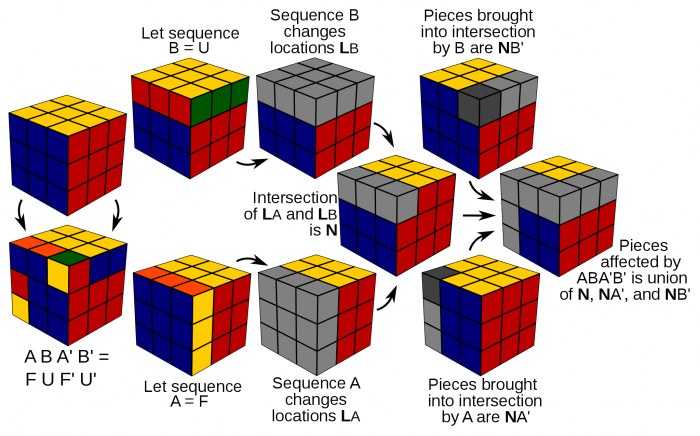Group Theory through Rubics Cubes (Source:  commons.wikimedia.org)

In the 19th Century, mathematicians were highly prolific, and many advances were made in terms of number theory:

• The law of quadratic reciprocity, which establishes links between the prime numbers (theorised by Euler and demonstrated by Gauss)
• Prime number theorem
• Proof of Fermat’s Last Theorem, notably by Ernst Kummer, who demonstrates this for any exponent of less than 100.

Gauss and Legendre found the method of least squares, a major advance in statistics, a branch of probabilities. Hermann Grassmann is the originator of what is now called linear algebra and the notion of a vector space.

Another mathematician, Urbain Le Verrier, discovered the presence of Neptune in our solar system and calculated its weight. His calculations allowed the discovery of previously unseen planets.

That century also marks the beginnings of electricity and electromagnetic theory with the discoveries of Gauss, Ampère and Maxwell.

During the same period, Albert Einstein demonstrated the law of cubic reciprocity, known as the Einstein Integer. One of the most enduring texts of the time remains Riemann’s 1859 Manuscript, in which the German mathematician explored what later became known as the Riemann Zeta Function, with a hypothesis that is still highly relevant today to areas like quantum mechanics and number theory.

The 20th century began with a list of 23 unsolved problems that were to occupy the minds of many a scientist and mathematician. This century is dominated by three mathematical theorems:

• Gödel’s incompleteness theorems, which address the question of coherence in mathematics (read more about undecidable statements)
• The Taniyama-Shimura Conjecture, also known as modality theorem, thanks to which Fermat’s last theorem is at last solved!
• The Weil conjectures on generating functions: Series whose coefficients encode sequences of numbers.

During the 20th century, the continuous evolution of maths and science gave rise to new fields, like topology, differential geometry and algebra.

Mechanics have been the subject of extensive study, famously by Einstein and Pointcarré, with the theory of general relativity.

Group Theory occupied many of the best minds in maths for several decades, culminating in a complete classification of finite simple groups, in 1980. Thanks to the automated calculations possible with computers, the Four colour theorem was also solved.

The 21st century has begun with advances such as great advances by maths prodigy Terence Tao on prime numbers and other areas.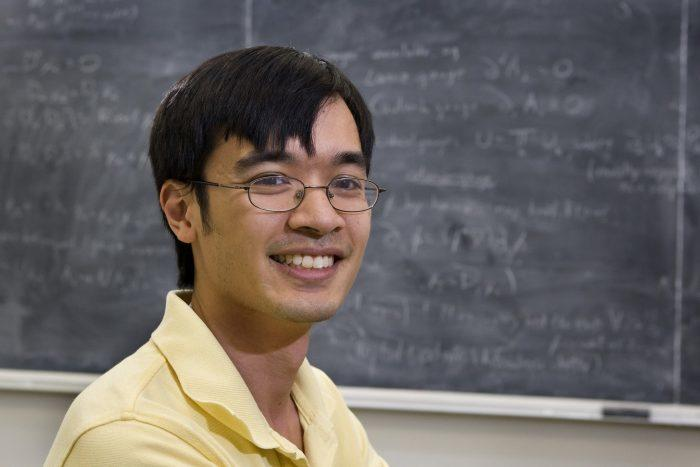Modern-day maths genius Terence Tao (Source: wikipedia.org)

On October 8, 2013, the Nobel Prize for Physics was jointly awarded to François Englert and Peter Higgs “for the theoretical discovery of a mechanism that contributes to our understanding of the origin of mass of subatomic particles..”.

In this case, fiction accurately predicted real life: Dr. Simon Singh, the British popular science author, in the 2013 book titled “The Simpsons And Their Mathematical Secrets” commenting on a scene in the famous cartoon in which Homer is working on a mathematical equation wrote:

“If you work it out, you get the mass of the Higgs Boson that’s only a bit larger than the nano-mass of a Higgs Boson actually is. It is kind of amazing as Homer makes the prediction 14 years before it was discovered”  “

Despite the great progress made over recent decades, rest assured that there are an infinite number of discoveries yet to be made! To learn more, about what we do know, read our blogs about:

Share(No ratings so far)Loading...